Test: Congruence Criteria- SAS And ASA

# Test: Congruence Criteria- SAS And ASA

Test Description

## 15 Questions MCQ Test Mathematics (Maths) Class 9 | Test: Congruence Criteria- SAS And ASA

Test: Congruence Criteria- SAS And ASA for Class 9 2022 is part of Mathematics (Maths) Class 9 preparation. The Test: Congruence Criteria- SAS And ASA questions and answers have been prepared according to the Class 9 exam syllabus.The Test: Congruence Criteria- SAS And ASA MCQs are made for Class 9 2022 Exam. Find important definitions, questions, notes, meanings, examples, exercises, MCQs and online tests for Test: Congruence Criteria- SAS And ASA below.
Solutions of Test: Congruence Criteria- SAS And ASA questions in English are available as part of our Mathematics (Maths) Class 9 for Class 9 & Test: Congruence Criteria- SAS And ASA solutions in Hindi for Mathematics (Maths) Class 9 course. Download more important topics, notes, lectures and mock test series for Class 9 Exam by signing up for free. Attempt Test: Congruence Criteria- SAS And ASA | 15 questions in 15 minutes | Mock test for Class 9 preparation | Free important questions MCQ to study Mathematics (Maths) Class 9 for Class 9 Exam | Download free PDF with solutions
 1 Crore+ students have signed up on EduRev. Have you?
Test: Congruence Criteria- SAS And ASA - Question 1

### In the given figure, AB = AC, AD = AE = 5 cm and DC = 8 cm. Length of EB is______.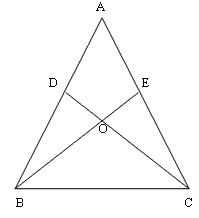Detailed Solution for Test: Congruence Criteria- SAS And ASA - Question 1

8 cm is the answer because DC = EB = 8 cm.

Test: Congruence Criteria- SAS And ASA - Question 2

### Triangle ABC is congruent to triangle DEF. Which side is congruent to side BC?

Detailed Solution for Test: Congruence Criteria- SAS And ASA - Question 2

The side BC is congruent to side EF.

Test: Congruence Criteria- SAS And ASA - Question 3

### Which of the following statements is incorrect ?

Detailed Solution for Test: Congruence Criteria- SAS And ASA - Question 3

2 rectangles having same area may differ in their lengths. So they will not be congruent

Test: Congruence Criteria- SAS And ASA - Question 4

In the following figure, PQ = PR and SQ = SR, then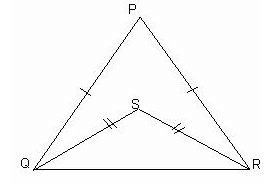Detailed Solution for Test: Congruence Criteria- SAS And ASA - Question 4

In △PQR and △SRQ,

► PQ = SR(Given)
► QR = QR(Common)
► PR = SQ(Given)

By SSS property:
△PQR≅△SRQ
Therefore, ∠PQS =∠PRS

Test: Congruence Criteria- SAS And ASA - Question 5

If two triangles ABC and PQR are congruent under the correspondence A ↔ P, B ↔ Q and C ↔ R, then symbolically, it is expressed as

Test: Congruence Criteria- SAS And ASA - Question 6

If ΔABC ≌ ΔPQR then, which of the following is true?

Detailed Solution for Test: Congruence Criteria- SAS And ASA - Question 6

Corresponding sides in congruent triangles are equal.

So AC = PR ,AB = PQ ,BC = QR

Test: Congruence Criteria- SAS And ASA - Question 7

If two sides of a triangle are equal, the angles opposite to these sides are______.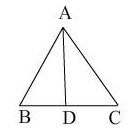Detailed Solution for Test: Congruence Criteria- SAS And ASA - Question 7

Theorem: Angles opposite to equal sides of an isosceles triangle are equal.

Test: Congruence Criteria- SAS And ASA - Question 8

In case of two equilateral triangles, PQR and STU which of the following correspondence is not correct?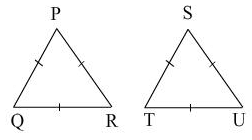Detailed Solution for Test: Congruence Criteria- SAS And ASA - Question 8

The correct option is Option A.

All equilateral triangles have the same angles by the congruence rule (SSS)

So, TTS <—> PQR

Test: Congruence Criteria- SAS And ASA - Question 9

In quadrilateral ADBC, AB bisects ∠A. Which of the following criterion will prove ΔABC ≅ ΔABD?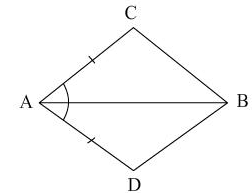Detailed Solution for Test: Congruence Criteria- SAS And ASA - Question 9

► AC = AD
and AB being the bisector of ∠A.

Now, in ΔABC and ΔABD:
► AC = AD     [Given]
► AB = AB     [Common]
► ∠CAB = ∠DAB [∴ AB bisects ∠CAD]

∴ Using SAS criteria, we have
ΔABC ≌ ΔABD.

∴ Corresponding parts of congruent triangles (c.p.c.t) are equal.
∴ BC = BD

Test: Congruence Criteria- SAS And ASA - Question 10

In an isosceles triangle ABC with AB = AC, if BD and CE are the altitudes, then BD and CE are______.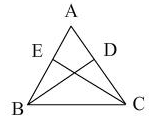Detailed Solution for Test: Congruence Criteria- SAS And ASA - Question 10

Given: △ABC, AB=AC, BD⊥AC and CE⊥AB
Area of triangle = 1/2 × base × height
Area of △ABC = 1/2 × ​AB × CE = 1/2 × ​BD × AC
CE = BD (Since, AB=AC)

Test: Congruence Criteria- SAS And ASA - Question 11

In fig., if AB = AC and PB = QC, then by which congruence criterion PBC ≅ QCB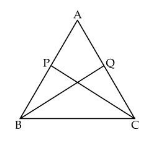Detailed Solution for Test: Congruence Criteria- SAS And ASA - Question 11

As AB = AC so angle ACB = angle(ABC) as angles opposite to equal sides r equal.

In triangle PBC and Triangle QCB we see that:
i) PB = QC (given)
ii) angle(PBC) = (angle)QCB (proved earliar)
iii) BC = BC (common)

So, triangle PBC is congruent to triangle QCB by SAS axiom of congruency.

Test: Congruence Criteria- SAS And ASA - Question 12

The diagonal PR of a quadrilateral PQRS bisects the angles P and R, then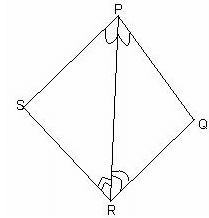Detailed Solution for Test: Congruence Criteria- SAS And ASA - Question 12

In Δ PSR and ΔPQR

► PR = PR
► ∠ 1 = ∠ 2
► ∠ 3 = ∠ 4

Δ PSR ≅ Δ PQR  [ASA]

► PS = PQ   [CPCT]
► QR = RS  [CPCT]

The correct option is Option A

Test: Congruence Criteria- SAS And ASA - Question 13

In the given figure, AB = EF, BC = DE, AB ⊥ BD and EF ⊥ CE. Which of the following criterion is true for ΔABD ≅ ΔEFC?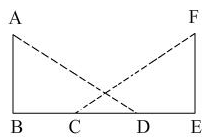Detailed Solution for Test: Congruence Criteria- SAS And ASA - Question 13

In triangle ABD and FEC:
► AB = FE ( given )
► ∠FEC = ∠ABD ( 90degree)
► BC = DE
CD is common part coming in both triangles.
► BC + CD = CD + DE
► BD = CE
Therefore, triangle ABD is congruent to triangle FEC by SAS rule of congruence.

Test: Congruence Criteria- SAS And ASA - Question 14

Two figures are congruent if they have______.

Detailed Solution for Test: Congruence Criteria- SAS And ASA - Question 14

In geometry, two figures or objects are congruent if they have the same shape and size, or if one has the same shape and size as the mirror image of the other.

Test: Congruence Criteria- SAS And ASA - Question 15

A triangle can have______.

Detailed Solution for Test: Congruence Criteria- SAS And ASA - Question 15

The least number of acute angles that a triangle can have is 2.

As we cannot have more than one right angle or obtuse angle, we have only two or three acute angles in a triangle.

Further, if one angle is acute, sum of other two angles is more than 900 and we cannot have two right angles or obtuse angles.

## Mathematics (Maths) Class 9

84 videos|352 docs|109 tests
 Use Code STAYHOME200 and get INR 200 additional OFF Use Coupon Code
Information about Test: Congruence Criteria- SAS And ASA Page
In this test you can find the Exam questions for Test: Congruence Criteria- SAS And ASA solved & explained in the simplest way possible. Besides giving Questions and answers for Test: Congruence Criteria- SAS And ASA, EduRev gives you an ample number of Online tests for practice

## Mathematics (Maths) Class 9

84 videos|352 docs|109 tests